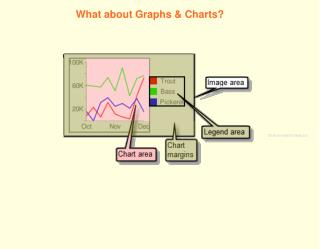DownloadDownload Presentation# What about Graphs & Charts?

Télécharger la présentation## What about Graphs & Charts?

- - - - - - - - - - - - - - - - - - - - - - - - - - - E N D - - - - - - - - - - - - - - - - - - - - - - - - - - -
##### Presentation Transcript

1. What about Graphs & Charts? Click on chart to fade out

2. Not sure about which graph to use? Confused between bar charts, line  graphs, and pie charts? Let's explore Charts.

3. A Line Graph Line graphs are used to track changes  over short and long periods of time. When smaller changes exist, line graphs  are better to use than bar graphs.

4. Let's analyze the data on this chart. Let's make a line graph.

5. A Bar Chart Bar charts are used to compare things  between different groups or to track changes  over time. However, when trying to measure change  over time, bar charts are best when the  changes are larger.

6. Let's analyze the data on this chart. Let's make a bar chart.

7. A Pie Chart Pie charts are best to use when you are  trying to compare parts of a whole. They do not show changes over time.

8. Let's analyze the data on this chart. Let's make a Pie Chart.

9. Venn Diagram The Venn Diagram is made up of two or more  overlapping circles. It is often used in  mathematics to show relationships between  sets. Venn Diagrams are useful for examining  similarities and differences. This activity enables students to organize  similarities and differences visually .

10. Let's analyze the data on this chart. Yellow Marbles Red Marbles Let's make a venn diagram. = 1 student Green Marbles

11. Pictograph A pictograph uses pictures or symbols to  represent an assigned amount of data. A pictograph takes the form of a bar  graph. The key for a pictograph tells the number  that each picture or symbol represents.

12. Let's analyze the data on this chart. Let's make a pictograph.

13. Nick, an analyst at a shipping company, reviewed historic  postal rates in order to predict future rate increases.

14. The students in Mr. Smith's class recorded the  number of siblings that each student has. How many students have at least 2 siblings? 3 7 12 23

15. Electoral Votes by States Use the Data in the tally chart to complete  the missing row in the pictograph. Each = 2 votes Click on Star for answer.

16. Which shape is at (3, 2)? 5 4 3 2 1 0 1 2 3 4 Push Button for answer.